×

Search anything:

# Contrast Enhancement Algorithms

#### Machine Learning (ML)Get this book -> Problems on Array: For Interviews and Competitive Programming

Contrast enhancement is an essential image processing technique which has applications in image processing where the subjective quality of images is important for human interpretation.

1. What is Contrast and Contrast Enhancement?
2. Types of Contrast Enhancement Algorithms:
• Histogram Equalization
• CLAHE
• Contrast Stretching
• Min-Max Contrast Stretching
3. Contrast Enhancement Algorithms in Python

# 1. What is Contrast and Contrast Enhancement?

Contrast refers to the amount of differentiation that is there between the various image features. Images having a higher contrast level generally display a greater degree of color or gray-scale variation than those of lower contrast.

Below shown is an example of a low contrast image and a high contrast image.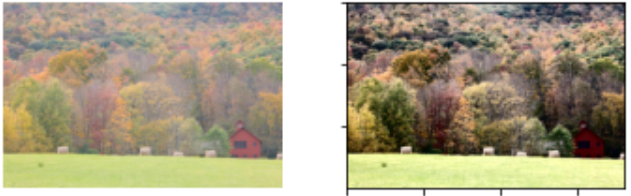In Section 3 you will learn how convert the low contrast image to a high contrast image yourself using not one but three algorithms!

Contrast Enhancement refers to the sharpening of image features to remove the noisy feature such as edges and contrast boundaries. Contrast Enhancement Algorithms aim to improve the perception of the image by human eye.

# 2. Types of Contrast Enhancement Algorithms:

## Histogram Equalization:

Histogram equalization is an image processing technique that adjusts image intensities to improve contrast. Histogram Equalization is one of the simplest and commonly used method in low level image enhancement using the histogram. The logic behind Histogram Equalization is that the image with the best visual appearance, is the one whose histogram looks like the regular distribution.

A Cumulative Distribution Function(CDF) of a histogram is the fraction of pixels in with an pixel value is less than or equal to the specified pixel value.

Histogram Equalization is particularyl useful in cases where both backgrounds and foregrounds are both bright or dark. Histogram Equalization can lead to better views of bone structure in x-ray images, and to better detail in over-exposed or under-exposed photos. A quintessential advantage of Histogram Equalization method is that it is a fairly straight forward image processing technique.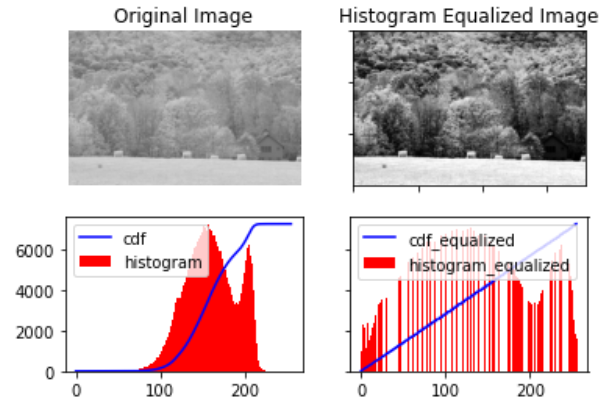As we can see from the image above, the image after Histogram Equalization is much easier to interpret. The Cumulative Distribution Function(CDF) of the image after Histogram Equalization ideally should be a straight line.

Contrast Enhancement of a Black and White image is fairly straight forward. Histogram Equalization of color images is a little complicated. OpenCV loads color images in BGR (Blue Green Red) color space. In BGR, it is not possible to perform histogram equalization without affecting the color information as all channels contain color information, therefore we have to convert the BGR image into YCrCb.
In YCrCb (Luminance; Chroma: Blue; Chroma: Red) color space, the Y channel of the image only contains intensity information where as Cr and Cb channels contain all the color information of the image. The operation mus tbe performed only only on the Y channel to get the Histogram Equalized output without changing any color related details.

The image before and after Histogram Equalization are shown below: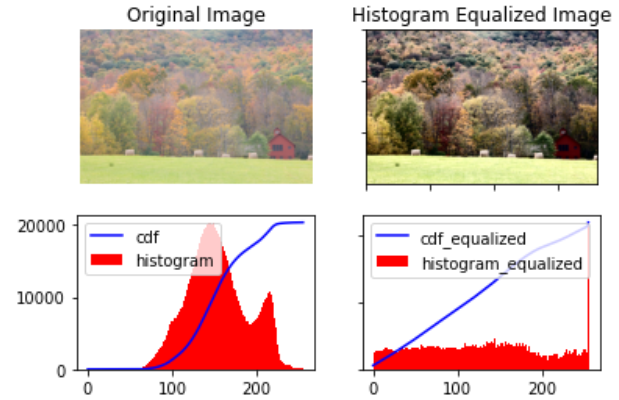Adaptive Histogram Equalization computes many histograms for each of the separate part of the image, and uses them to redistribute the lightness values of the image, hence it differs from Histogram Equalization. Hence it is suitable for bettering the local contrast in images.

### CLAHE:

Contrast Limited Adaptive Histogram Equalization(CLAHE) is a variant of Adaptive Histogram Equalization. CLAHE has one additional step over Adaptive Histogram Equalization and that is clipping of the histogram. The 5 steps in CLAHE are mentioned below:

• Divide the image into tiny regions.
• Decide the mapping functions of local histogram.
• Choose the clipping point of histogram.
• Apply the function to every region.
• Reduce the noise by the background subtraction method.

The image before and after CLAHE are shown below: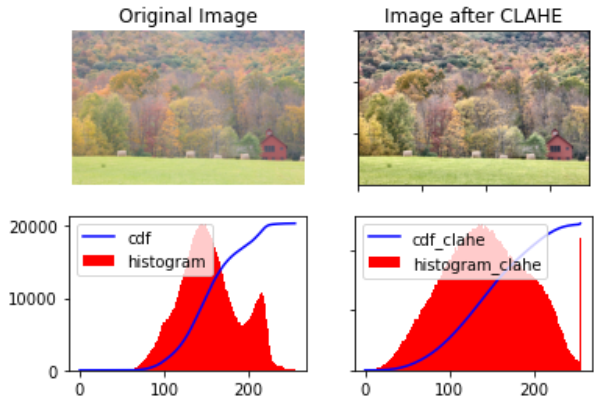## Contrast Stretching:

In Contrast Stretching the contrast in an image is stretched from the range of intensity values it contains to span a desired range of values.It is also called Normalization.

Some Contrast Stretching techniques include: Minimum-Maximum, Percentage, and Piecewise Contrast Enhancement.

### Min-Max Contrast Stretching:

In Min-Max Contrast Stretching for each pixel:

pixel = ((pixel – min) / (max – min))*255

Where min and max are the maximum and minimum pixel values in the image.

Below shown is an image before and after Min-Max Contrast Stretching: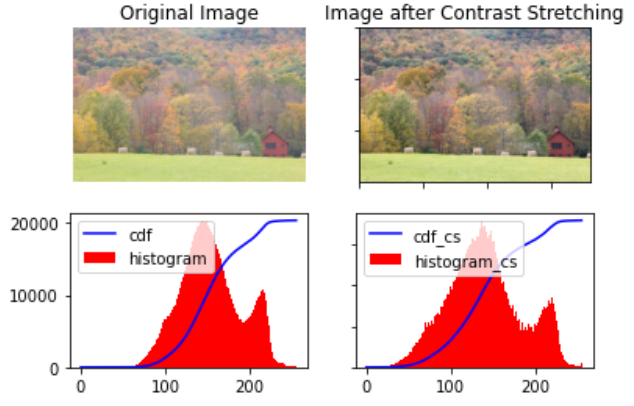# 3. Contrast Enhancement Algorithms in Python

## Histogram Equalization:

Histogram Equalization of a Black and White Image is fairly straight forward, and can be done using the hist_equalized function of OpenCV.

Importing the libraries

``````import numpy as np
import matplotlib.pyplot as plt
import cv2
import matplotlib.image as mpimg
``````

Reading the image and generating histograms and CDFs of the original image

``````# Reading the original image, here 0 implies that image is read as grayscale

# Generating the histogram of the original image
hist,bins = np.histogram(image.flatten(),256,[0,256])

# Generating the cumulative distribution function of the original image
cdf = hist.cumsum()
cdf_normalized = cdf * hist.max()/ cdf.max()
``````

Applying Histogram Equalization, and generating histograms and CDFs of the equalized image

``````# Applying Histogram Equalization on the original image
image_equalized = cv2.equalizeHist(image)

# Generating the histogram of the equalized image
hist_equalized,bins_equalized = np.histogram(image_equalized.flatten(),256,[0,256])

# Generating the cumulative distribution function of the original image
cdf_equalized = hist_equalized.cumsum()
cdf_equalized_normalized = cdf_equalized * hist_equalized.max()/ cdf_equalized.max()

``````

As mentioned in Section 2, to apply Histogram Equalization to color images we have to first convert then to the YCrCb color space. We will apply Histogram Equalization to just the Y channel and the code to do the same is mentioned below:

``````# Converting the image to YCrCb
image_yuv = cv2.cvtColor(image_c, cv2.COLOR_BGR2YUV)

# Applying Histogram Equalization on the original imageof the Y channel
image_yuv[:,:,0] = cv2.equalizeHist(image_yuv[:,:,0])
``````

## Contrast Limited Adaptive Histogram Equalization(CLAHE):

All of the other steps such as importing, creation of Histograms and CDFs, and application to just the Y channel in color images is the same in CLAHE as in Histogram Equalization the steps that vary are mentioned below:

``````#Creating CLAHE
clahe = cv2.createCLAHE(clipLimit=2, tileGridSize=(8,8))

#Apply CLAHE to the original image
image_clahe = clahe.apply(image)
``````

## Min-Max Contrast Stretching:

All of the other steps such as importing, creation of Histograms and CDFs, and application to just the Y channel in color images is the same in CLAHE as in Histogram Equalization the steps that vary are mentioned below:

Applying Min-Max Constrast Stretching instead of equalize_hist():

``````# Create an empty array to store the final output
image_cs = np.zeros((image.shape,image.shape),dtype = 'uint8')

# Apply Min-Max Contrasting
min = np.min(image)
max = np.max(image)

for i in range(image.shape):
for j in range(image.shape):
image_cs[i,j] = 255*(image[i,j]-min)/(max-min)
``````

To futher explain all the code in this article I have created Jupyter Notebooks which can be viewed here:

Hope that you found this article informative and understood what Contrast and Contrast Enhancement are, what are the different Contrast Enhancement Algorithms and how to implement them in Python using OpenCV.